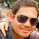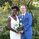561 görüntülenme
The 2 Pole Butterworth Filter was created by John Ehlers (Cycle Analytics For Traders pg 32) and this is an updated version of his original 2 pole Butterworth Filter script that seems to follow the price even closer. Buy when the indicator line turns green and sell when it turns red.

Let me know if there are other scripts you would like to see me publish or if you want something custom done!

## YorumlarHi Cheatcountry, amazing stuff. One question can this be applied to Forex? And since I am a rookie at this care to elaborate on how I can install this.
Cevap GönderPrime117
@Prime117, Yes this can be applied to any financial data
Cevap GönderpleasantCardin94082
@pleasantCardin94082, np. There are quite a few changes actually. Different input for one thing and different methods to calculate the coefficient values. It was Ehlers idea to multiply the square root by 1.25 and this eliminates some of the noise
Cevap GönderChakku
@Chakku, looks great. I would love to see the script behind this chart
Cevap Göndercheatcountry
Hi Cheatcountry, Sure it is an honour.

//@version=4
// Copyright (c) 2019-present, Franklin Moormann (cheatcountry)
study("Ehlers 2 Pole Butterworth Filter V2 ", overlay=true)

inp = input(title="Source", type=input.source, defval=close)
res = input(title="Resolution", type=input.resolution, defval="")
rep = input(title="Allow Repainting?", type=input.bool, defval=false)
bar = input(title="Allow Bar Color Change?", type=input.bool, defval=false)
src = security(syminfo.tickerid, res, inp)
length1 = input(title="Length", type=input.integer, defval=10, minval=1)
length2 = input(title="Length", type=input.integer, defval=15, minval=1)
length3 = input(title="Length", type=input.integer, defval=45, minval=1)
length4 = input(title="Length", type=input.integer, defval=50, minval=1)

pi = 2 * asin(1)

a1 = exp(-1.414 * pi / length1)
a2 = exp(-1.414 * pi / length2)
a3 = exp(-1.414 * pi / length3)
a4 = exp(-1.414 * pi / length4)

b1 = 2 * a1 * cos(1.414 * 1.25 * pi / length1)
b2 = 2 * a2 * cos(1.414 * 1.25 * pi / length2)
b3 = 2 * a3 * cos(1.414 * 1.25 * pi / length3)
b4 = 2 * a4 * cos(1.414 * 1.25 * pi / length4)

cx1 = b1
cx2 = b2
cx3 = b3
cx4 = b4

cy1 = -a1 * a1
cy2 = -a2 * a2
cy3 = -a3 * a3
cy4 = -a4 * a4

c1 = 1 - cx1 - cy1
c2 = 1 - cx2 - cy2
c3 = 1 - cx3 - cy3
c4 = 1 - cx4 - cy4

bf1 = 0.0
bf2 = 0.0
bf3 = 0.0
bf4 = 0.0

bf1 := (c1 * src) + (cx1 * nz(bf1)) + (cy1 * nz(bf1))
bf2 := (c2 * src) + (cx2 * nz(bf2)) + (cy2 * nz(bf2))
bf3 := (c3 * src) + (cx3 * nz(bf3)) + (cy3 * nz(bf3))
bf4 := (c4 * src) + (cx4 * nz(bf4)) + (cy4 * nz(bf4))

//sig1 = src > bf1 ? 1 : src < bf1 ? -1 : 0

RED = #fd1206
BLUE = #0a1cf9

//bfColor1 = sig1 > 0 ? BLUE : sig1 < 0 ? RED : color.black
bfColor1 = bf1 > bf1 ? BLUE :bf1 < bf1 ? RED : color.black
bfColor2 = bf2 > bf2 ? BLUE :bf2 < bf2 ? RED : color.black
bfColor3 = bf3 > bf3 ? BLUE :bf3 < bf3 ? RED : color.black
bfColor4 = bf4 > bf4 ? BLUE :bf4 < bf4 ? RED : color.black